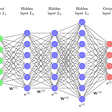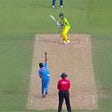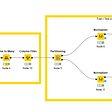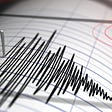# Data Overview

`This database contains 14 attributes(features).The "goal" field refers to the presence of heart disease in the patient. It is integer valued from 0 (no presence) to 4.Target: In the end we will classify given data in the range 0 to 4 clas of diease.`

# The performance metric for our model:

• Both precision and recall is important
• Confusion matrix

# Simple Intuition of the whole article

1. Importing all the needed libraries
3. Exploratory Data Analysis
4. Data Processing
5. Applying Machine learning model like (SVM, DT, KNN, XGBOOST, LR, RF)
6. Feature Importance
7. Comparing all the model (log loss).
8. Conclusion

# 1. Import libraries

`import numpy as npimport pandas as pdimport matplotlib.pyplot as pltfrom matplotlib import rcParamsfrom matplotlib.cm import rainbow%matplotlib inlineimport warningswarnings.filterwarnings('ignore')import plotly.graph_objs as goimport plotly.tools as tlsimport osimport gcimport refrom sklearn.model_selection import train_test_splitfrom sklearn.preprocessing import StandardScalerfrom sklearn.neighbors import KNeighborsClassifierfrom sklearn.svm import SVCfrom sklearn.tree import DecisionTreeClassifierfrom sklearn.ensemble import RandomForestClassifier`

`dataset=pd.read_csv('../input/heart-disease-uci/heart.csv')dataset.head()`
`dataset.info()<class 'pandas.core.frame.DataFrame'>RangeIndex: 303 entries, 0 to 302Data columns (total 14 columns):age         303 non-null int64sex         303 non-null int64cp          303 non-null int64trestbps    303 non-null int64chol        303 non-null int64fbs         303 non-null int64restecg     303 non-null int64thalach     303 non-null int64exang       303 non-null int64oldpeak     303 non-null float64slope       303 non-null int64ca          303 non-null int64thal        303 non-null int64target      303 non-null int64dtypes: float64(1), int64(13)memory usage: 33.3 KB`

# 3. Exploratory Data Analysis

`print('~> Have not heart disease (target = 0):\n   {}%'.format(100 - round(dataset['target'].mean()*100, 2)))print('\n~> Have heart disease (target= 1):\n   {}%'.format(round(dataset['target'].mean()*100, 2)))~> Have not heart disease (target = 0):   45.54%~> Have heart disease (target= 1):   54.46%rcParams['figure.figsize'] = 20, 14plt.matshow(dataset.corr())plt.yticks(np.arange(dataset.shape), dataset.columns)plt.xticks(np.arange(dataset.shape), dataset.columns)plt.colorbar()`
`plt.figure(figsize=(6,6))dataset.groupby("target").count().plot.bar()`
`dataset.hist()`
`import seaborn as snssns.pairplot(dataset, palette='rainbow')`
`import seaborn as snsplt.figure(figsize=(12, 8))plt.subplot(1,2,1)sns.violinplot(x = 'target', y = 'chol', data = dataset[0:])plt.show()`
`sns.lmplot(x='chol',y='target',data=dataset)`
`plt.figure(figsize=(5, 5))unique_variations = dataset['chol'].value_counts()print('Number of Unique chol:', unique_variations.shape)# the top 10 variations that occured mostprint(unique_variations.head(10))s = sum(unique_variations.values);h = unique_variations.values/s;plt.plot(h, label="Histrogram of Variations")plt.xlabel('Index of a chol')plt.ylabel('Number of Occurances')plt.legend()plt.grid()plt.show()c = np.cumsum(h)print(c)plt.figure(figsize=(5, 5))plt.plot(c,label='Cumulative distribution of Variations')plt.grid()plt.legend()plt.show()Number of Unique chol: 152234    6204    6197    6269    5212    5254    5226    4243    4240    4239    4Name: chol, dtype: int64`
`rcParams['figure.figsize'] = 8,6plt.bar(dataset['target'].unique(), dataset['target'].value_counts(), color = ['red', 'green'])plt.xticks([0, 1])plt.xlabel('Target Classes')plt.ylabel('Count')plt.title('Count of each Target Class')`

# 4. Data Processing

`nan_rows = dataset[dataset.isnull().any(1)]print (nan_rows)Empty DataFrameColumns: [age, sex, cp, trestbps, chol, fbs, restecg, thalach, exang, oldpeak, slope, ca, thal, target]Index: []`
`categorical_feature_mask = dataset.dtypes==object# filter categorical columns using mask and turn it into alistcategorical_cols = dataset.columns[categorical_feature_mask].tolist()print(categorical_cols)print("number of categorical features ",len(categorical_cols))[]number of categorical features  0`
`standardScaler = StandardScaler()columns_to_scale = ['age', 'trestbps', 'chol', 'thalach', 'oldpeak']dataset[columns_to_scale] = standardScaler.fit_transform(dataset[columns_to_scale])`

# 5. Applying Machine Learning Model

`y = dataset['target']X = dataset.drop(['target'], axis = 1)X_train, X_test, y_train, y_test = train_test_split(X, y, test_size = 0.33, random_state = 0)`

# Performance metrics

`# This function plots the confusion matrices given y_i, y_i_hat.def plot_confusion_matrix(test_y, predict_y):    C = confusion_matrix(test_y, predict_y)    # C = 9,9 matrix, each cell (i,j) represents number of points of class i are predicted class j        A =(((C.T)/(C.sum(axis=1))).T)            B =(C/C.sum(axis=0))      plt.figure(figsize=(20,4))        labels = [1,2]    # representing A in heatmap format    cmap=sns.light_palette("blue")    plt.subplot(1, 3, 1)    sns.heatmap(C, annot=True, cmap=cmap, fmt=".3f", xticklabels=labels, yticklabels=labels)    plt.xlabel('Predicted Class')    plt.ylabel('Original Class')    plt.title("Confusion matrix")        plt.subplot(1, 3, 2)    sns.heatmap(B, annot=True, cmap=cmap, fmt=".3f", xticklabels=labels, yticklabels=labels)    plt.xlabel('Predicted Class')    plt.ylabel('Original Class')    plt.title("Precision matrix")        plt.subplot(1, 3, 3)    # representing B in heatmap format    sns.heatmap(A, annot=True, cmap=cmap, fmt=".3f", xticklabels=labels, yticklabels=labels)    plt.xlabel('Predicted Class')    plt.ylabel('Original Class')    plt.title("Recall matrix")        plt.show()`

## 5.1 K Neighbors Classifier

`knn_scores = []for k in range(1,21):    knn_classifier = KNeighborsClassifier(n_neighbors = k)    knn_classifier.fit(X_train, y_train)    knn_scores.append(knn_classifier.score(X_test, y_test))`
`from sklearn.calibration import CalibratedClassifierCVfrom sklearn.linear_model import SGDClassifierfrom sklearn.metrics import confusion_matrixfrom sklearn.metrics.classification import accuracy_score, log_lossplt.figure(figsize=(8,5))plt.plot([k for k in range(1, 21)], knn_scores, color = 'red')for i in range(1,21):    plt.text(i, knn_scores[i-1], (i, knn_scores[i-1]))plt.xticks([i for i in range(1, 21)])plt.xlabel('Number of Neighbors (K)')plt.ylabel('Scores')plt.title('K Neighbors Classifier scores for different K values')#############we get k best value that is 8#now again train our modelclf=KNeighborsClassifier(n_neighbors=14)clf.fit(X_train,y_train)clf = CalibratedClassifierCV(clf, method="sigmoid")clf.fit(X_train, y_train)predict_y=clf.predict_proba(X_test)print("The log loss is:",log_loss(y_test, predict_y, labels=clf.classes_, eps=1e-15))#########Plot confusion atrixprint(len(predict_y))#print(len(y_test))plot_confusion_matrix(y_test, clf.predict(X_test))The log loss is: 0.4194913074929284100`

## 5.2 Support Vector Classifier

`svc_scores = []kernels = ['linear', 'poly', 'rbf', 'sigmoid']for i in range(len(kernels)):    svc_classifier = SVC(kernel = kernels[i])    svc_classifier.fit(X_train, y_train)    svc_scores.append(svc_classifier.score(X_test, y_test))`
`colors = rainbow(np.linspace(0, 1, len(kernels)))plt.bar(kernels, svc_scores, color = colors)for i in range(len(kernels)):    plt.text(i, svc_scores[i], svc_scores[i])plt.xlabel('Kernels')plt.ylabel('Scores')plt.title('Support Vector Classifier scores for different kernels')##################### with best kernel clf=SVC(kernel ='rbf')#clf.fit(X_train,y_train)clf = CalibratedClassifierCV(clf, method="sigmoid")clf.fit(X_train, y_train)predict_y=clf.predict_proba(X_test)print("The log loss is:",log_loss(y_test, predict_y, labels=clf.classes_, eps=1e-15))#########Plot confusion atrixprint(len(predict_y))#print(len(y_test))plot_confusion_matrix(y_test, clf.predict(X_test))The log loss is: 0.4152790470358795100`

## 5.3 Decision Tree Classifier

`dt_scores = []for i in range(1, len(X.columns) + 1):    dt_classifier = DecisionTreeClassifier(max_features = i, random_state = 0)    dt_classifier.fit(X_train, y_train)    dt_scores.append(dt_classifier.score(X_test, y_test))`
`plt.plot([i for i in range(1, len(X.columns) + 1)], dt_scores, color = 'green')for i in range(1, len(X.columns) + 1):    plt.text(i, dt_scores[i-1], (i, dt_scores[i-1]))plt.xticks([i for i in range(1, len(X.columns) + 1)])plt.xlabel('Max features')plt.ylabel('Scores')plt.title('Decision Tree Classifier scores for different number of maximum features')clf=DecisionTreeClassifier(max_features = 10, random_state = 0)clf.fit(X_train,y_train)clf = CalibratedClassifierCV(clf, method="sigmoid")clf.fit(X_train, y_train)predict_y=clf.predict_proba(X_test)print("The log loss is:",log_loss(y_test, predict_y, labels=clf.classes_, eps=1e-15))#########Plot confusion atrixprint(len(predict_y))#print(len(y_test))plot_confusion_matrix(y_test, clf.predict(X_test))The log loss is: 0.5341998825924514100`

## 5.4 Random Forest Classifier

`rf_scores = []estimators = [10, 100, 200, 500, 1000]for i in estimators:    rf_classifier = RandomForestClassifier(n_estimators = i, random_state = 0)    rf_classifier.fit(X_train, y_train)    rf_scores.append(rf_classifier.score(X_test, y_test))`
`colors = rainbow(np.linspace(0, 1, len(estimators)))plt.bar([i for i in range(len(estimators))], rf_scores, color = colors, width = 0.8)for i in range(len(estimators)):    plt.text(i, rf_scores[i], rf_scores[i])plt.xticks(ticks = [i for i in range(len(estimators))], labels = [str(estimator) for estimator in estimators])plt.xlabel('Number of estimators')plt.ylabel('Scores')plt.title('Random Forest Classifier scores for different number of estimators')clf=RandomForestClassifier(n_estimators = 200, random_state = 0)clf.fit(X_train,y_train)clf = CalibratedClassifierCV(clf, method="sigmoid")clf.fit(X_train, y_train)predict_y=clf.predict_proba(X_test)print("The log loss is:",log_loss(y_test, predict_y, labels=clf.classes_, eps=1e-15))#########Plot confusion atrixprint(len(predict_y))#print(len(y_test))plot_confusion_matrix(y_test, clf.predict(X_test))The log loss is: 0.39348441062524186100`

## 5.5 XGBOOST -ensemble technique

`import xgboost as xgbparams = {}params['objective'] = 'binary:logistic'params['eval_metric'] = 'logloss'params['eta'] = 0.02params['max_depth'] = 4d_train = xgb.DMatrix(X_train, label=y_train)d_test = xgb.DMatrix(X_test, label=y_test)watchlist = [(d_train, 'train'), (d_test, 'valid')]bst = xgb.train(params, d_train, 400, watchlist, early_stopping_rounds=20, verbose_eval=10)xgdmat = xgb.DMatrix(X_train,y_train)predict_y = bst.predict(d_test)print("The test log loss is:",log_loss(y_test, predict_y, labels=clf.classes_, eps=1e-15))print(len(predict_y))#print(len(y_test))plot_confusion_matrix(y_test, clf.predict(X_test))	train-logloss:0.681559	valid-logloss:0.684108Multiple eval metrics have been passed: 'valid-logloss' will be used for early stopping.Will train until valid-logloss hasn't improved in 20 rounds.	train-logloss:0.585605	valid-logloss:0.60957	train-logloss:0.512725	valid-logloss:0.557567	train-logloss:0.455003	valid-logloss:0.519062	train-logloss:0.407503	valid-logloss:0.49514	train-logloss:0.368371	valid-logloss:0.478558	train-logloss:0.336583	valid-logloss:0.466736	train-logloss:0.308998	valid-logloss:0.460353	train-logloss:0.285831	valid-logloss:0.453601	train-logloss:0.265688	valid-logloss:0.448218	train-logloss:0.248369	valid-logloss:0.441384	train-logloss:0.233118	valid-logloss:0.438462	train-logloss:0.219548	valid-logloss:0.43408	train-logloss:0.208009	valid-logloss:0.434458	train-logloss:0.197807	valid-logloss:0.43351	train-logloss:0.189129	valid-logloss:0.43454Stopping. Best iteration:	train-logloss:0.203719	valid-logloss:0.432805The test log loss is: 0.43494783621281385100`

## 5.6 Logistic Regression

`alpha = [10 ** x for x in range(-5, 2)] # hyperparam for SGD classifier.log_error_array=[]for i in alpha:    clf = SGDClassifier(alpha=i, penalty='l2', loss='log', random_state=42)    clf.fit(X_train, y_train)    sig_clf = CalibratedClassifierCV(clf, method="sigmoid")    sig_clf.fit(X_train, y_train)    predict_y = sig_clf.predict_proba(X_test)    log_error_array.append(log_loss(y_test, predict_y, labels=clf.classes_, eps=1e-15))    print('For values of alpha = ', i, "The log loss is:",log_loss(y_test, predict_y, labels=clf.classes_, eps=1e-15))fig, ax = plt.subplots()ax.plot(alpha, log_error_array,c='g')for i, txt in enumerate(np.round(log_error_array,3)):    ax.annotate((alpha[i],np.round(txt,3)), (alpha[i],log_error_array[i]))plt.grid()plt.title("Cross Validation Error for each alpha")plt.xlabel("Alpha i's")plt.ylabel("Error measure")plt.show()best_alpha = np.argmin(log_error_array)clf = SGDClassifier(alpha=alpha[best_alpha], penalty='l2', loss='log', random_state=42)clf.fit(X_train, y_train)sig_clf = CalibratedClassifierCV(clf, method="sigmoid")sig_clf.fit(X_train, y_train)predict_y = sig_clf.predict_proba(X_train)print('For values of best alpha = ', alpha[best_alpha], "The train log loss is:",log_loss(y_train, predict_y, labels=clf.classes_, eps=1e-15))predict_y = sig_clf.predict_proba(X_test)print('For values of best alpha = ', alpha[best_alpha], "The test log loss is:",log_loss(y_test, predict_y, labels=clf.classes_, eps=1e-15))predicted_y =np.argmax(predict_y,axis=1)print("Total number of data points :", len(predicted_y))plot_confusion_matrix(y_test, predicted_y)For values of alpha =  1e-05 The log loss is: 0.44012529267994355For values of alpha =  0.0001 The log loss is: 0.42826340471379937For values of alpha =  0.001 The log loss is: 0.4560308526539795For values of alpha =  0.01 The log loss is: 0.41489190299443834For values of alpha =  0.1 The log loss is: 0.40163904302879466For values of alpha =  1 The log loss is: 0.40556761746085845For values of alpha =  10 The log loss is: 0.4124918369135891`
`For values of best alpha =  0.1 The train log loss is: 0.37686236801831313For values of best alpha =  0.1 The test log loss is: 0.40163904302879466Total number of data points : 100`

# 6. Feature Importance

`clf=RandomForestClassifier(n_estimators = 500, random_state = 0)clf.fit(X_train,y_train)features = X_train.columnsimportances = clf.feature_importances_indices = (np.argsort(importances))[-25:]plt.figure(figsize=(10,12))plt.title('Feature Importances')plt.barh(range(len(indices)), importances[indices], color='r', align='center')plt.yticks(range(len(indices)), [features[i] for i in indices])plt.xlabel('Relative Importance')plt.show()`

# 7. Comparing Models

`error_rate=np.array([0.3828,0.4158,0.3972,0.5409,0.3803,0.4135])plt.figure(figsize=(16,5))print(error_rate)#plt.scatter(error_rate,range(1,7))#seed = 7# prepare modelsmodels = ['LR','XGBOOST','RF','DT','SVM','KNN']plt.xlabel(models)plt.plot(error_rate)lowest_loss=np.argmin(error_rate)print("lowest logg loss : ",min(error_rate))print(models[lowest_loss])[0.3828 0.4158 0.3972 0.5409 0.3803 0.4135]lowest logg loss :  0.3803SVM`

# 8.Conclusion

--

--

--

## More from Akash Kumar

Software Engineer 👨‍💻

Love podcasts or audiobooks? Learn on the go with our new app.

## Techniques of Feature Selection in Machine Learning## Natural Language Processing Anyone Can Use## A Guide to Text Annotation — the Key to Understanding Language## A Start-to-Finish Guide to Building Deep Neural Networks in Keras## WomanLife: Deep Learning for the detection and classification of breast cancer## Automatic Deep Learning hyper Parameters search strategy## It’s Deep Learning Times: A New Frontier of Data## Step-by-Step Deep Learning Tutorial to Build your own Video Classification Model## Akash Kumar

Software Engineer 👨‍💻

## Hiring Prediction using AI / ML## Churn Analysis with Feature Selection -Genetic Algorithm## Credit Card Transactions Fraud Prediction with Machine Learning Algorithms## LANL Earthquake Prediction